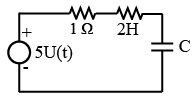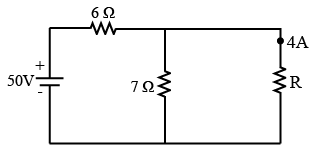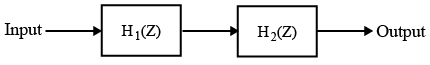# ISRO Scientist or Engineer Electronics 2006

Instructions

For the following questions answer them individually

Question 1

# The value of C which gives the critical damping in the given circuit isQuestion 2

# A series RLC circuit resonates at 3 MHz and has 3-dB bandwidth of 10 kHz. The Q of the circuit at resonanceQuestion 3

# The value of resistance R shown in the given figure isQuestion 4

# At 3-dB frequencies, current in the series RLC circuit equal current at resonance multiplied byQuestion 5

# A series RLC circuit resonates at 1000 kHz. At frequency of 995 kHz, the circuit impedance isQuestion 6

# If each stage had gain of 10dB and noise figure of 10dB, then the overall noise figure of two-stage cascade amplifier will beQuestion 7

# In Sigma-delta ADC, high bit accuracy is achieved byQuestion 8

# Let s(t) denote the delta function. The value of the integral$$\int_{-a}^{a}\delta(t) \cos \left(\frac{3t}{2}\right)dt$$ isQuestion 9

# Consider the compound system shown in the above figure. Its output is equal to the input with a delay of two units. If the transfer function of the first system is given by $$H_1(Z) = \frac{Z - 0.5}{Z - 0.8}$$, then theQuestion 10

# The z-transform of the signal$$x(n) = \begin{cases}1, & n = -1\\2, & n = 0 \\ -1, & n = 1 \\1, & n = 2 \\0, & otherwise \end{cases}$$OR